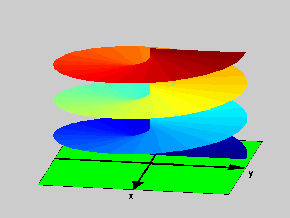18.04 | Fall 1999 | Undergraduate

# Complex Variables with Applications

Study Materials

## Riemann Surfaces: The Logarithm

(All images created with MATLAB® software)

Click on picture for more information and an image of higher resolution.

Riemann Surfaces provide a nice way to visualize multiple valued functions in the complex plane. In fact: on its Riemann Surface a multiple valued function is single valued, it is only when the Riemann Surface is projected on the Complex Plane that the multiple values arise. However: what is the Riemann Surface for a multiple valued function and how is it defined? We will not even attempt to be rigorous here, but just give the general idea (followed by several examples). It goes as follows:

Consider a multiple valued function and all it’s branches (corresponding to some fixed set of cuts). Think of all these branches as sort of “Lego” set with which the surface will be constructed.  As we pointed out earlier, these various branches will be linked: when a branch cut is approached and crossed in one of them, the natural continuation of the values of the function will correspond to some other branch. Thus, we can take all the various copies of the cut complex planes on which the branches are defined (as single valued functions) and then “join” them across the cuts according to their correspondences (each “lip” of a cut will go to a different branch). This will then produce a surface on which the function is defined as single valued in a smooth way (no discontinuities anywhere). This surface is the Riemann Surface.

For example, in the case of the logarithm, we can start with the complex plane cut along the negative real axis. On this cut plane, the logarithm has infinitely many branches, each differing from the “next” by 2πι. Thus, if we join all these cut planes (lower lip of the cut(*1) in a plane to the upper lip of the cut in the prior plane), what we obtain is the infinite spiral staircase-like surface displayed on the picture.

Notice that the only singularity in this surface occurs at the location of the branch point. This is a general feature of Riemann Surfaces.

(*1) By lower lip and upper lip, we mean here the sides of the cut along the negative real axis that correspond to a negative (respectively, positive) imaginary part.Figure 1: Riemann Surface: The Logarithm